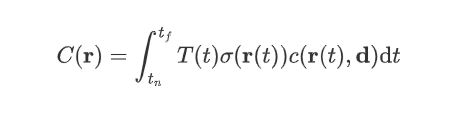## Part. 1 翻译

*使用带位姿信息的神经辐射场表示场景

### 摘要

NeRF 使用一个全连接的深度网络表示场景，输入为连续的 5D 坐标，输出为该位置的体积密度和视角相关的辐射强度。

NeRF 方法通过查询射线的 5D 坐标合成视角，使用经典的体积渲染技术输出颜色和密度，投影到图像中。体积渲染可导，通过这一点可以优化表示，使得只需要使用一组有已知相机姿态的图像作为输入。

### 1. 引言

（总之，我们提出了一种高质量且高性能的神经渲染方法）

1. 沿相机射线生成一系列 3D 采样点。
2. 使用对应的 2D 观察方向作为输入，生成一组输出颜色和密度。
3. 使用体积渲染技术将输出积累到 2D 图像中，可以使用梯度下降法优化。

### 2. 相关工作

NeRF 增强了神经场景表示的能力，以产生渲染复杂逼真场景的最先进结果。

### 4. 辐射场体渲染

5D 神经辐射场将场景表示为空间中各个点的体密度和方向辐射强度。使用经典体积渲染原理来渲染穿过场景的任意射线的颜色。

$$C({\bold r})=\int_{t_n}^{t_f}T(t)\sigma({\bold r}(t))c({\bold r}(t),{\bold d}){\rm d}t$$

，其中 $T(t)=\exp{(-\int_{t_n}^t\sigma(\bold r(s)){\rm d}s)}$.

$$t_i\sim u\begin{bmatrix}t_n+{{i-1}\over N}(t_f-t_n),t_n+{i\over N}(t_f-t_n)\end{bmatrix}.$$

，其中 $\delta_i=t_{i+1}-t_i$，为分层取样点的间距。

### 5. 优化神经辐射场

（1）对输入坐标进行位置编码，帮助 MLP 表示高频率函数。

（2）使用层次体积抽样。

## Part. 2 问题

### 摘要

1. 什么是体积渲染技术？

体积渲染即神经渲染，相较于原来的光栅化/光线追踪，使用神经网络进行逆向渲染.

### 辐射场体渲染

1. 公式 $(3)$ 中的 $u$ 是什么？

$u$ 指代均匀分布

2. MLP 连续评估的原理？

3. 文中所指的 alpha 合成是什么？好像并不是前景合成透明图片？

## Part. 3 理解分析

NeRF 使用的重要概念之一，神经辐射场，其数据形式即 $(c,\sigma)$，使用如下模型隐式表达：$T(t)=\exp{(-\int_{t_n}^t\sigma(\bold r(s)){\rm d}s)}$，$r(t)=o+t_d$.

MLP 倾向于学习低频信息，导致渲染表示颜色和几何图形的高频变化方面表现得比较差。作者还表明，在将输入传递到网络之前，高频函数可以将输入映射到更高维空间，更好你和包含高频变化的数据。